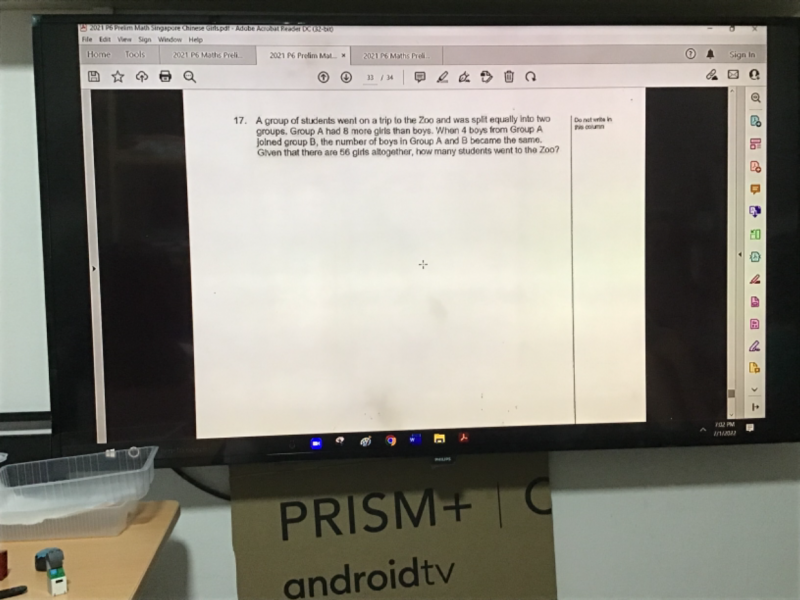# QuestionPls help to solve thx

<—-   Girls  ——–><- Boys—>

Group A       [ ——–u———][  u – 4  ]{4}        where {4}  is minus away

Group B       [————2u + 8 —————-](4)   where (4) is added on

(u+8)  + (2u+8)+ 4 (u-4) = 56

2u + 24 = 56

2u = 32 => u = 16

Total students who went to the zoo(Group A + Group B) = 2(2u + 8) = 2[2(16) + 8] = 80